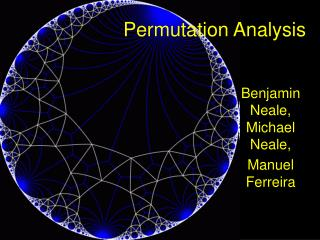Download PresentationPermutation Analysis

# Permutation Analysis - PowerPoint PPT PresentationDownload Presentation## Permutation Analysis

- - - - - - - - - - - - - - - - - - - - - - - - - - - E N D - - - - - - - - - - - - - - - - - - - - - - - - - - -
##### Presentation Transcript

1. Permutation Analysis Benjamin Neale, Michael Neale, Manuel Ferreira

2. Who came up with permutation? • Hint: it’s a statistical tool • R. A. Fisher • Proposed as validation for Student’s t-test in 1935 in Fisher’s The Design of Experiments

3. Basic Principle • Under the null, all data comes from the same distribution • We calculate our statistic, such as mean difference • We then shuffle the data with respect to group and recalculate the statistic (mean difference) • Repeat step 3 multiple times • Find out where our statistic lies in comparison to the null distribution

4. Real Example • Case-Control data, and we want to find out if there is a mean difference Mean difference .541

5. Permutation One Mean difference = .329

6. Simulation example • I simulated 70 data points from a single distribution—35 cases and 35 controls • Mean difference of -.21 • I then permuted randomly assigning case or control status • Empirical significance=#hits/#permutations

7. Empirical Significance • #hits is any permuted dataset that had a mean difference >.21 or <-.21 • #permutations is the trials permuted datasets we generate • Result(#hits/#permutations) = 2024/5000 = .4048 • T test results = .3672

8. General principles of permutation • Disrupt the relationship being tested • Mean difference between group: switch groups • Test for linkage in siblings: randomly reassign the ibd sharing • If matched control then within pair permute • Shaun will describe tests for association

9. General advantages • Does not rely on distributional assumptions • Corrects for hidden selection • Corrects for hidden correlation

10. How would we do QTL permutation in Mx? • We analyze our real data and record χ2 • For sibpairs we shuffle the ibd probabilities for each sibpair • We reanalyze the data and record the new χ2 • We generate a distribution of χ2 for the permuted sets • Place our statistic on the distribution • Repeat for all locations in genome

11. Some caveats • Computational time can be a challenge • Determining what to maintain and what to permute • Variable pedigrees also pose some difficulties • Need sufficient data for combinations • Unnecessary when no bias, but no cost to doing it

12. Some Exercises • What would we permute to determine if the MZ correlation is equal to the DZ correlation in a univariate analysis? • What would we permute to determine if QTL dominance is significant in linkage analysis